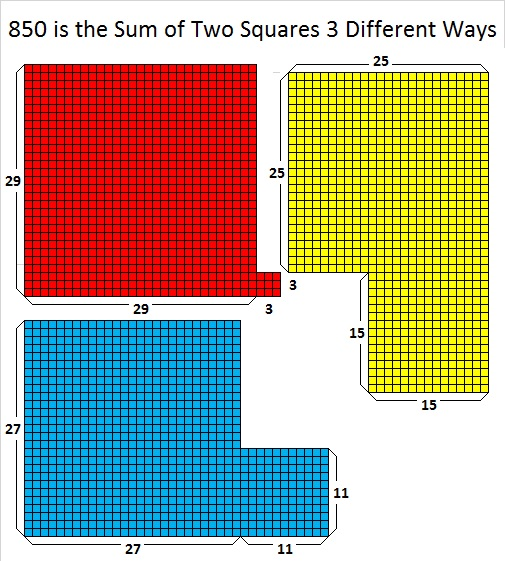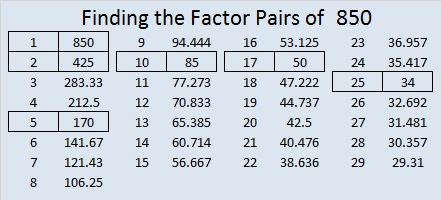# How Often is 850 the Hypotenuse of a Pythagorean Triple?

We can tell if a number is the hypotenuse of a Pythagorean triple by looking at its prime factorization.

• If NONE of its prime factors leave a remainder of 1 when divided by 4, then it will NOT be the hypotenuse of Pythagorean triple.
• If at least one of its prime factors leave a remainder of 1 when divided by 4, then it WILL be the hypotenuse of Pythagorean triple.
• If ALL of its prime factors leave a remainder of 1 when divided by 4, then it will also be the hypotenuse of at least one PRIMITIVE Pythagorean triple.

850 = 2 × 5² × 17¹. Its factor, 2, prevents 850 from being the hypotenuse of a primitive Pythagorean triple, but 5² × 17¹ will actually make it the hypotenuse of SEVEN Pythagorean triples. Some of those we can find by looking at the ways we can make 850 from the sum of two squares:29² + 3² = 850, 27² + 11² = 850, and 25² + 15² = 850

• 29² + 3² gives us 174-832-850, calculated from 2(29)(3), 29² – 3², 29² + 3², and is 2 times (87-416-425)
• 27² + 11² gives us 594-608-850, calculated from 27² – 11², 2(27)(11), 27² + 11², and is 2 times (297-304-425)
• 25² + 15² gives us 400-750-850 calculated from 2(25)(15), 25² – 15², 25² + 15², and is (8-15-17) times 50.

Let’s look a little closer at 850’s factoring information:

• 850 is a composite number.
• Prime factorization: 850 = 2 × 5 × 5 × 17, which can be written 850 = 2 × 5² × 17
• The exponents in the prime factorization are 1, 2, and 1. Adding one to each and multiplying we get (1 + 1)(2 + 1)(1 + 1) = 2 × 3 × 2 = 12. Therefore 850 has exactly 12 factors.
• Factors of 850: 1, 2, 5, 10, 17, 25, 34, 50, 85, 170, 425, 850
• Factor pairs: 850 = 1 × 850, 2 × 425, 5 × 170, 10 × 85, 17 × 50, or 25 × 34
• Taking the factor pair with the largest square number factor, we get √850 = (√25)(√34) = 5√34 ≈ 29.154759.Here are the SEVEN ways 850 is the hypotenuse of a Pythagorean triple with five of 850’s factor pairs in bold print:

• 174-832-850 which is 2 times (87-416-425)
• 594-608-850 which is 2 times (297-304-425)
• 510-680-850 which is (3-4-5) times 170.
• 130-840-850 which is 10 times (13-84-85).
• 360-770-850 which is 10 times (36-77-85).
• 400-750-850 which is (8-15-17) times 50
• 238-816-850 which is(7-24-25) times 34

When I wrote about 845, I said I would explore a conjecture a little more:

My conjecture: If prime numbers x and y are Pythagorean triple hypotenuses, and A and B are integers with B ≥ A and A ≥ 1, then xᴬ × y will have two primitive triples. The total number of triples xᴬ × yᴮ will have will be A + B + 2Bᴬ

So…how many Pythagorean triples does 2 × 5³ × 17¹ = 4250 have? It will have the same number as 5³ × 17¹ = 2125.

From the conjecture I figure that 2125 and 4250 will each have 1 + 3 + 2(3¹) = 10 total triples. Let’s see if I’m right …

Besides 1 and itself, the factors of 2125 are 5, 17, 25, 85, 125, and 425, all Pythagorean triple hypotenuses. Each of their respective primitive Pythagorean triples has a multiple with 4250 as the hypotenuse:

1. 850 times 5’s primitive
2. 250 times 17’s primitive
3. 175 times 25’s primitive
4. 34 times 125’s primitive
5. 50 times 85’s two primitives
6. 10 times 425’s two primitives

That’s a total of 8 Pythagorean triples from that list. We will also have triples that are 2 times 2125’s primitives. We can find those triples by looking at the sums of two squares that equal 4250.

1. 65² + 5² = 4250; but 5 is a factor of both 65 and 5, so this will produce a duplicate of one of the triples already given.
2. 61² + 23² = 4250; gives us 2806-3192-4250, calculated from 2(61)(23), 61² – 23², 61² + 23²
3. 55² + 35² = 4250; but 5 is a factor of both 55 and 35, so this will produce a duplicate of one of the triples already given.
4. 49² + 43² = 4250; gives us 552-4214-4250, calculated from 49² – 43², 2(49)(43), 49² + 43²

That gives us 2 more triples to add to the previous 8 for a total of 10 Pythagorean triples, and my conjecture still holds true.

Now one more thing about the number 850, here’s how to write it in a couple other bases:

• 505 BASE 13, because 5(13²) + 5(1) = 5(170) = 850.
• PP BASE 33 (P is 25 base 10) because 25(33) + 25(1) = 25(34) = 850